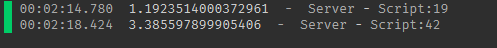# Distance between Vector3's is more performant than Vector2's?

After testing which is more performant (dist between vector3’s or vector2’s), I found that calculating between vector3’s is much more performant.

I’m confused why? Since there’s another axis, meaning more numbers to calculate, shouldnt vector2’s be faster? Is something with my benchmark code wrong?

``````task.wait(3)

-- Vector3

local a = Vector3.new(34.654, 546.654, 546.654)
local b = Vector3.new(54365.65464, 654.645, 546.654)

local function dist()
for i = 1, 8 do
local dist = (a - b).Magnitude
end
end

local start = os.clock()

for i = 1, 4_000_000 do
dist()
end

print(os.clock() - start)

-----------

-----------

-- Vector2 (why is this less performant?)

local a = Vector2.new(34.654, 546.654)
local b = Vector2.new(54365.65464, 654.645)

local function dist2()
for i = 1, 8 do
local dist = (a - b).Magnitude
end
end

local start = os.clock()

for i = 1, 4_000_000 do
dist2()
end

print(os.clock() - start)
``````The reason why is because Vector2 requires more stack frames then a Vector3

1 Like
1 Like

This topic was automatically closed 14 days after the last reply. New replies are no longer allowed.